How Cheenta works to ensure student success?
Explore the Back-Story

# RMO2018 Tamil Nadu Solutions and Problems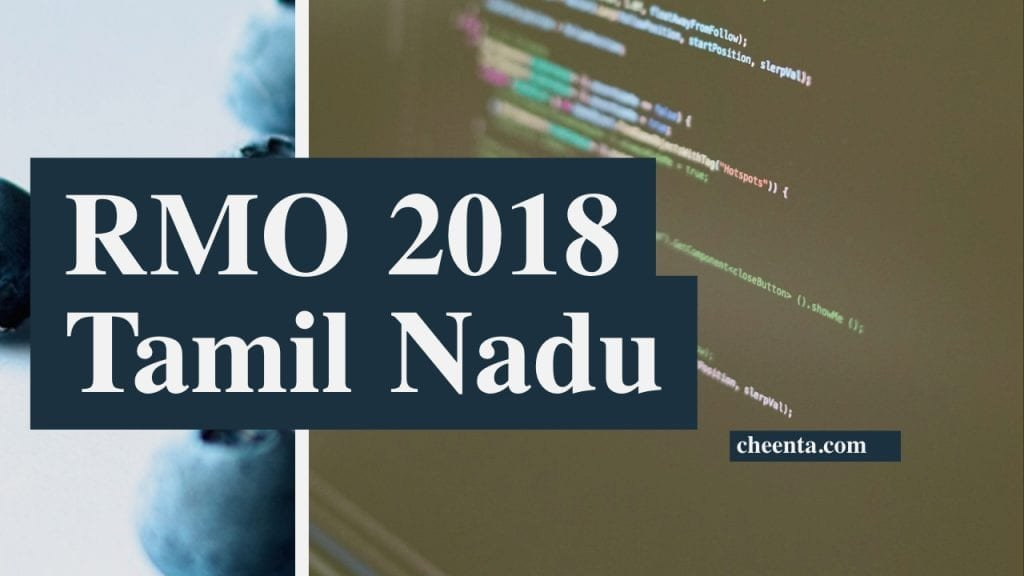RMO2018 Tamil Nadu Solutions (Sequential Hints) and related discussion follows. This is a work in progress.

Also see

1. Let ABC be an acute-angled triangle and let D be an interior point of the line segment BC. Let the circumcircle of triangle ACD intersect AB at E (E between A and B) and let the circumcircle of triangle ABD intersect AC at F (F between A and C). Let O be the circumcenter triangle AEF. Prove that OD bisects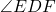2. Find the set of all real values of a for which the real polynomial equation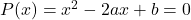has real roots, given that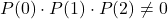andform a geometric progression.
3. Show that there are infinitely many 4-tuples (a, b, c, d) of natural numbers such that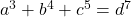.
4. Suppose 100 points in the plane are colored using two colors, red and white, such that each red point is the center of a circle passing through at least three white points. What is the least possible number of white points?
5. In a cyclic quadrilateral ABCD with circumcenter O, the diagonals AC and BD intersect at X. Let the circumcircles of triangles AXD and BXC intersect at Y. Let the circumcircles of triangle AXB and CXD intersect at Z. If O lies inside ABCD and if the points O, X, Y, Z are all distinct, prove that O, X, Y, Z lie in a circle.
6. Define a sequence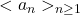of real numbers by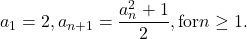Prove that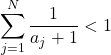for every natural number N.

You may also like: RMO 2018 (All India)

RMO2018 Tamil Nadu Solutions (Sequential Hints) and related discussion follows. This is a work in progress.

Also see

1. Let ABC be an acute-angled triangle and let D be an interior point of the line segment BC. Let the circumcircle of triangle ACD intersect AB at E (E between A and B) and let the circumcircle of triangle ABD intersect AC at F (F between A and C). Let O be the circumcenter triangle AEF. Prove that OD bisects2. Find the set of all real values of a for which the real polynomial equationhas real roots, given thatandform a geometric progression.
3. Show that there are infinitely many 4-tuples (a, b, c, d) of natural numbers such that.
4. Suppose 100 points in the plane are colored using two colors, red and white, such that each red point is the center of a circle passing through at least three white points. What is the least possible number of white points?
5. In a cyclic quadrilateral ABCD with circumcenter O, the diagonals AC and BD intersect at X. Let the circumcircles of triangles AXD and BXC intersect at Y. Let the circumcircles of triangle AXB and CXD intersect at Z. If O lies inside ABCD and if the points O, X, Y, Z are all distinct, prove that O, X, Y, Z lie in a circle.
6. Define a sequenceof real numbers byProve thatfor every natural number N.

You may also like: RMO 2018 (All India)

This site uses Akismet to reduce spam. Learn how your comment data is processed.

### Knowledge Partner